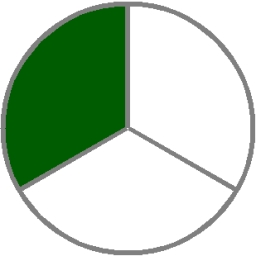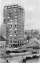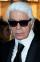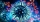# One third

If 3/5 is 360, how much is 1/3?

y =  200

### Step-by-step explanation:Did you find an error or inaccuracy? Feel free to write us. Thank you!Tips to related online calculators
Need help to calculate sum, simplify or multiply fractions? Try our fraction calculator.
Do you have a linear equation or system of equations and looking for its solution? Or do you have a quadratic equation?

## Related math problems and questions:

• FlourKim needs 3/4 cup of flour to make 12 cookies. How much flour would she need to make 60 cookies?
• Hotel 4A 360 room hotel has 1/3 of its rooms occupied at present . How many rooms are empty?
• Lenka and moneyWhen Lenka bought pants from 1/3 of her savings shirt from 1/5 and 1/8 spent at the hairdresser, she is left 328 Kč. How much money was saved? How much hairdresser cost?
• EmployeesOf all 360 employees, there are 11/12 women. How many men work in a company?
• Fraction of a NumberIf 1/2 of 1/3 of 1/4 of 1/5 of a number is 2.5. what is the number?
• Birthday partyFor her youngest son's birthday party, the mother bought 6 3/4 kg of hotdog and 5 1/3 dozens bread rolls. Hotdogs cost 160 per kilogram, and a dozen bread rolls cost 25. How much did she spend in all?
• Eq-fracSolve the following equation with fractions: h + 1/3 =5/3
• Fractions and mixed numerals(a) Convert the following mixed numbers to improper fractions. i. 3 5/8 ii. 7 7/6 (b) Convert the following improper fraction to mixed number. i. 13/4 ii. 78/5 (c) Simplify these fractions to their lowest terms. i. 36/42 ii. 27/45 2. evaluate following ex
• Trees3/5 trees are apples, cherries are 1/3. 5 trees are pear. How many is the total number of trees?
• If-then equationIf 1/2 + 2/5s = s - 3/4 what is the value of s?
• Dividing moneyMilan divided 360 euros in the ratio 4: 5, Erik in the ratio 500: 625. How many euros did the individual parts of Milan and how many Erik?
• What is 9What is the value of x in the proportion 2 and one-fourth over x = 1 and one-half over 3 and three-fifths? 2 and two-fifths 5 and two-fifths 8 and 1 over 10 12 and 3 over 20
• Evaluate expressionEvaluate expression using BODMAS rule: 1 1/4+1 1/5÷3/5-5/8
• TeacherTeacher Rem bought 360 pieces of cupcakes for the outreach program of their school. 5/9 of the cupcakes were chocolate flavor and 1/4 wete pandan flavor and the rest were a vanilla flavor. How much more pandan flavor cupcakes than vanilla flavor?3 3/4 + 2 3/5 + 5 1/2 Show your solution.Sum of two fractions is 4 3/7. If one of the fractions is 2 1/5 find the other one .2/3x + 5/7 = 1/2x + 22/21